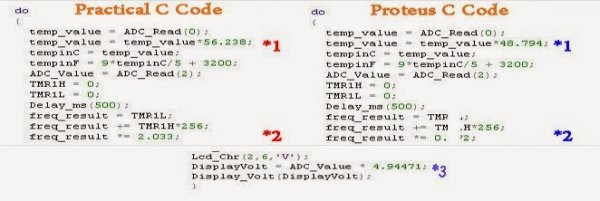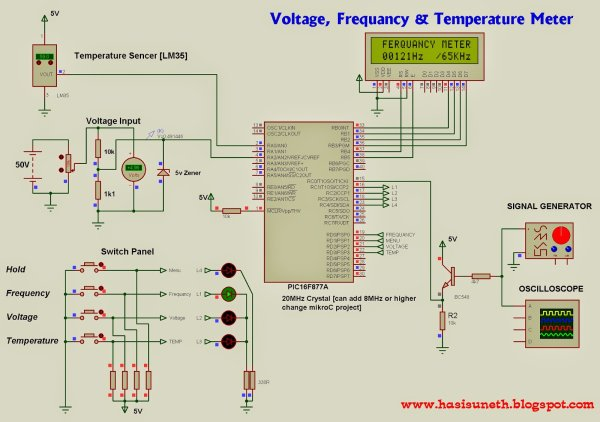# Voltage, Temperature & Frequency Meter With PIC Micro controller

Features…

• Voltage, Temperature and Frequency can switching to each others using push buttons.
• Voltage Meter can measure DC voltage between 0v to 50v.
• Frequency Meter can measure frequency up to 65KHz.
• Temperature Meter can use between 0C to 150C.
• Every functions can paused using push button.Instructions…

Voltage meter –

Volt meter can measure dc voltages up to 50v. 5V is the maximum voltage can handle PIC microcontroller, as it is voltage divider (10K,1.1K) use for convert 50V to 5V . 5v zener diode use for safety of PIC microcontroller analog input pin.

Temperature meter –

Temperature meter can use between 0C to 150C. But LM35 sensor can use between -55C to 150C. Sensor’s resistant is changing opposite to temperature, as it is maximum temperature gives maximum voltage output(5v)  and minimum temperature gives minimum voltage output(0v).

Frequency meter –

Theoretically, frequency meter can use up to 65KHz, that is because this circuit made by using Timer 1(16bit) of PIC microcontroller.Timer 0 can only measure up to 256Hz, that is because timer 0 is 8 bit timer. Transistor part is use for safety of microcontroller from amplitude of wave signal. This circuit can apply 12v amplitude or more changing transistor base resistor value(4.7K). I tested it, using 12v amplitude square wave signal

Troubleshooting…

If you have problem about accuracy of voltmeter, temperature meter and frequency meter, you can change values (*1,*2,*3)

For more detail: Voltage, Temperature & Frequency Meter With PIC Micro controller

#### Current Project / Post can also be found using:

• temperature measurement project
• frequency counter using 8*2 lcd circuit pdf
• frequency meter proteus pic
• pic sine wave ferequeny meter with microc